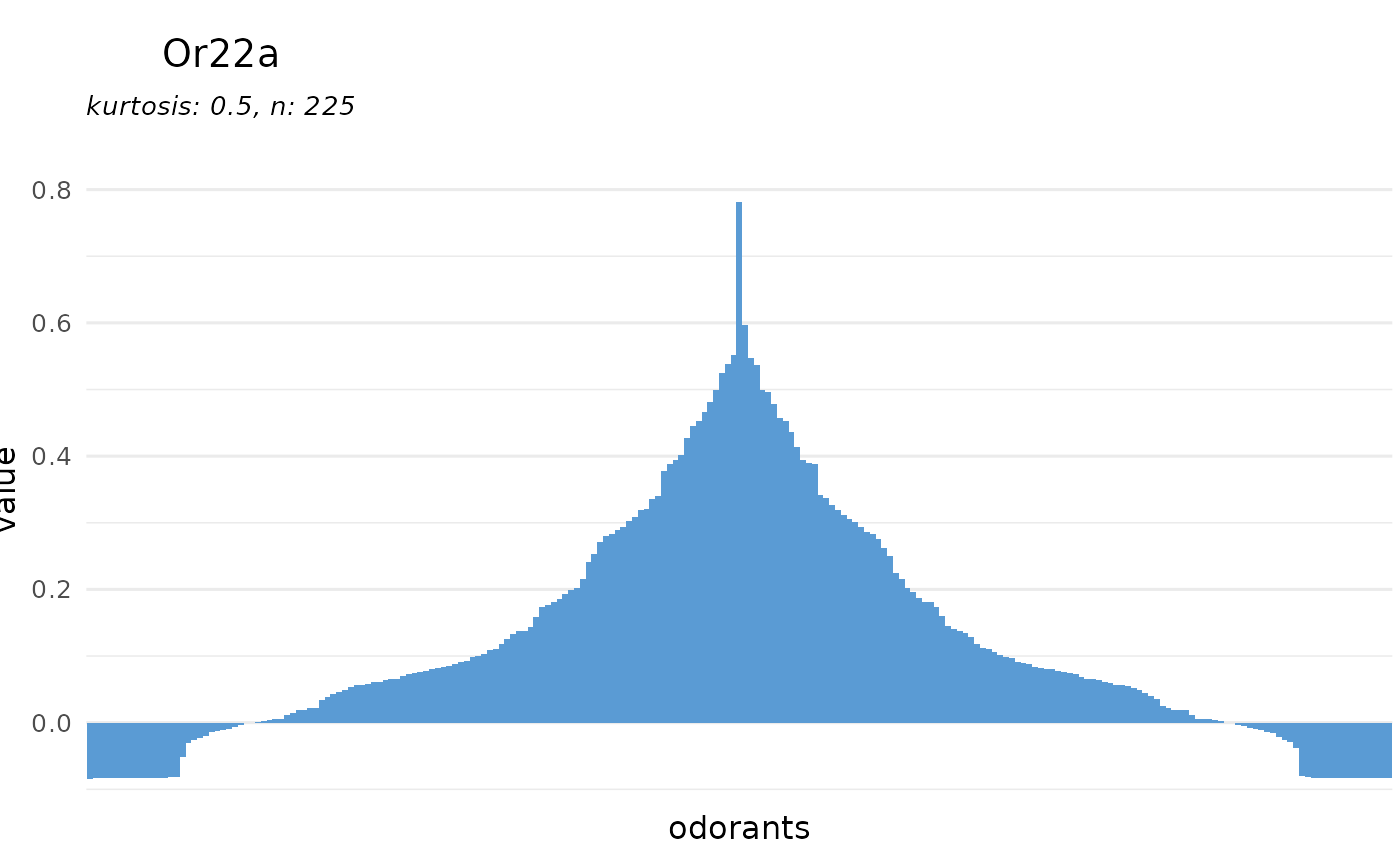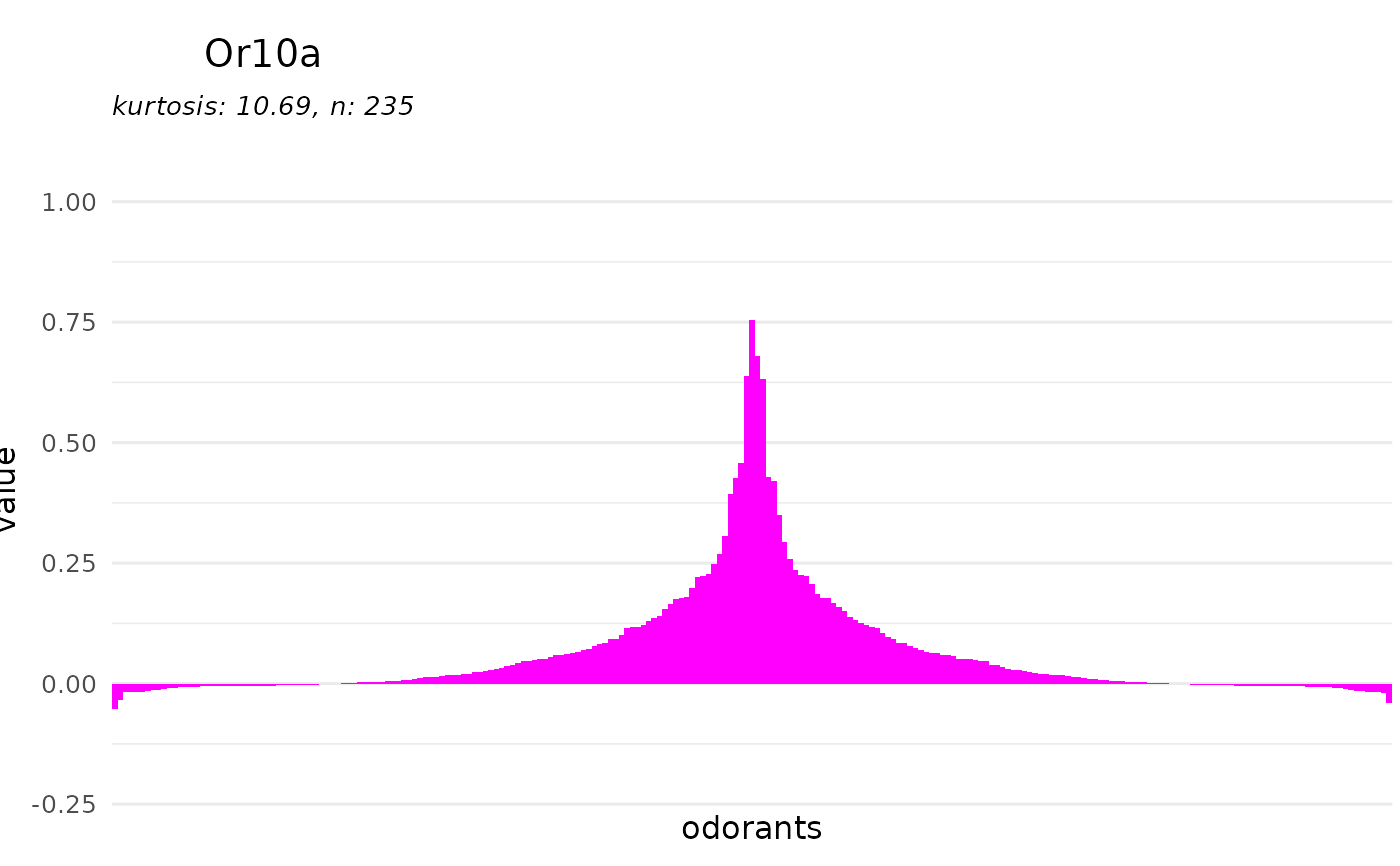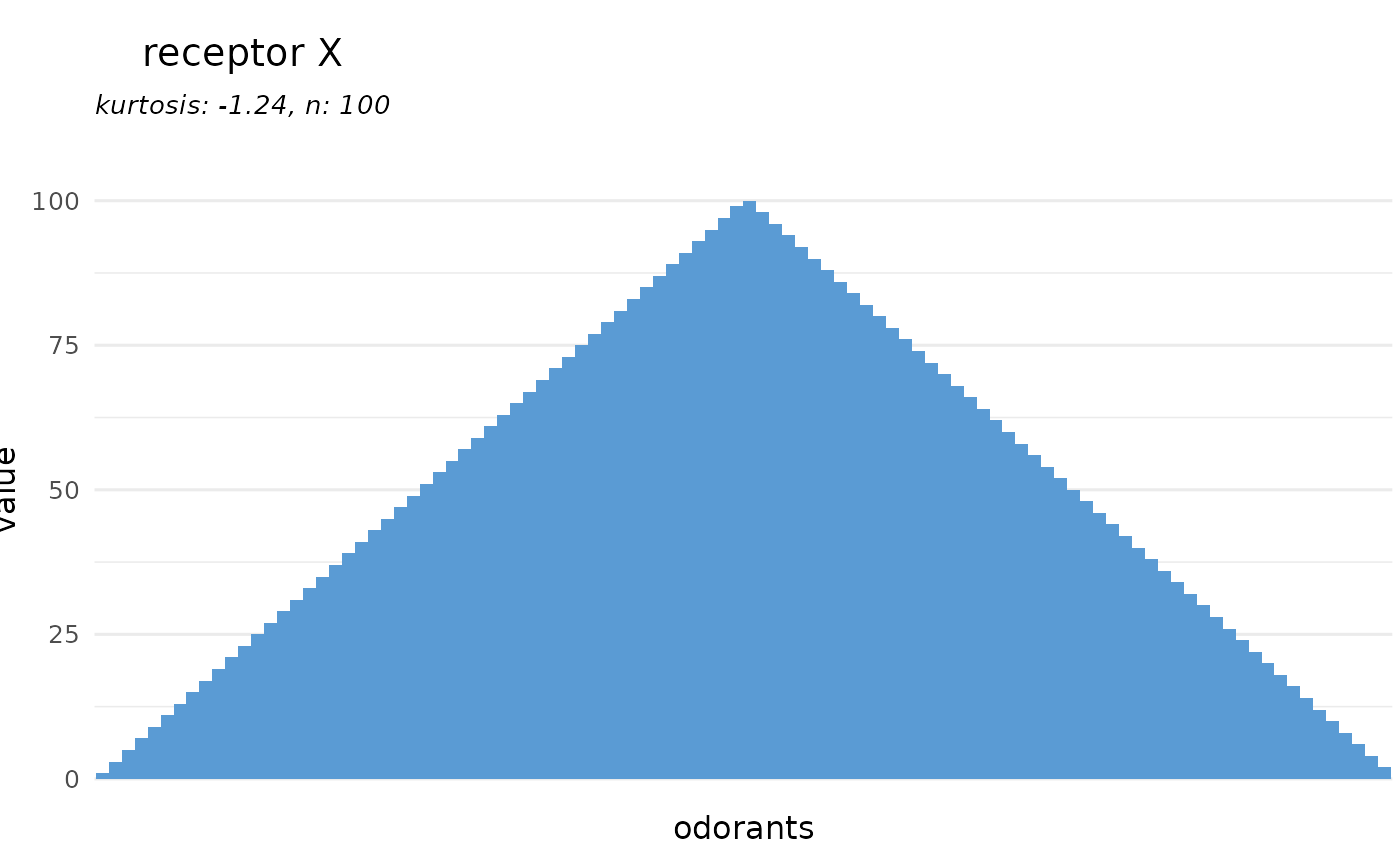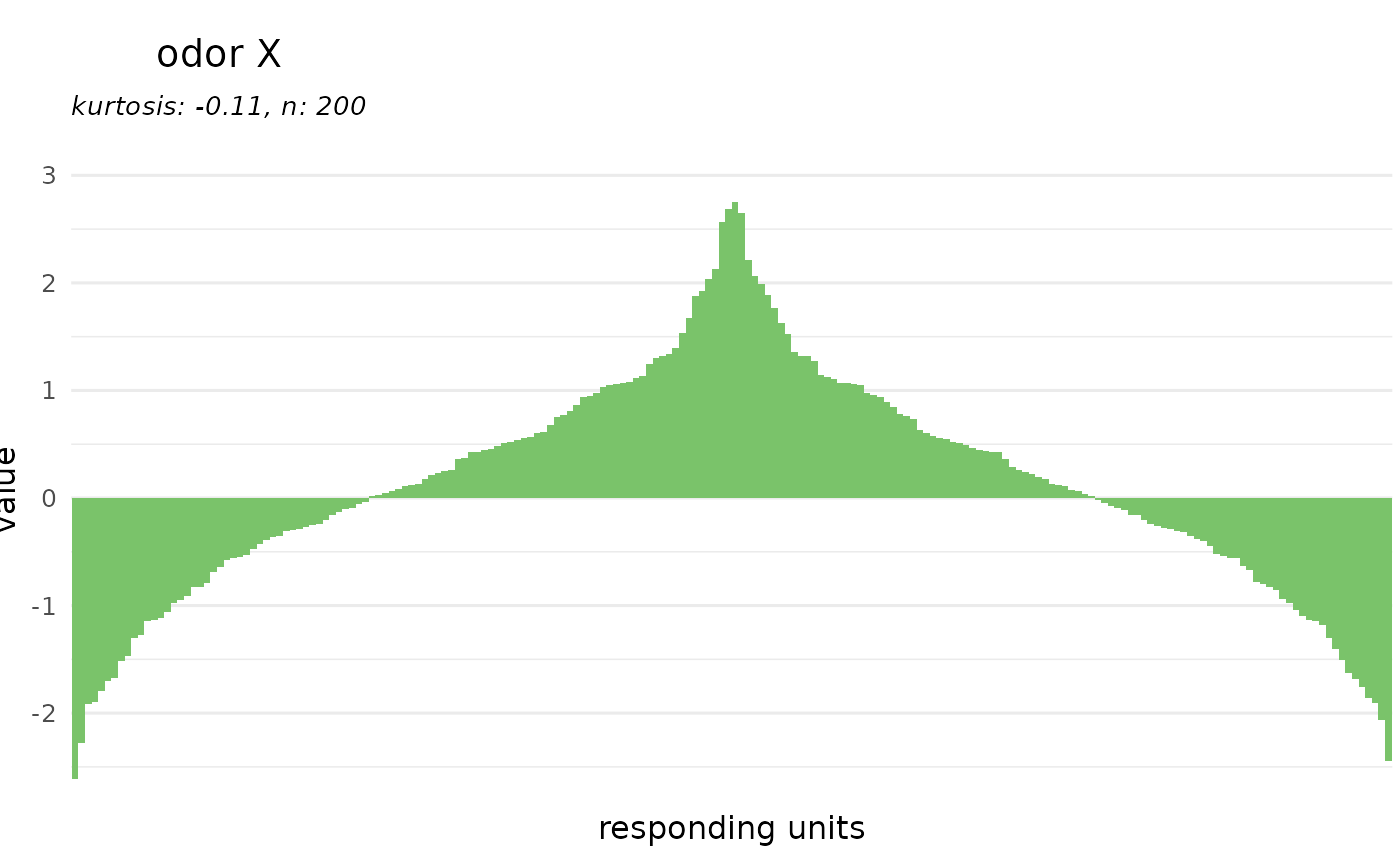plot a receptor or odorant tuning curve

## Usage

``````dplot_tuningCurve(receptor, odorant, response.vector,
response_matrix = door_default_values("door_response_matrix"),
odor_data = door_default_values("odor"),
zero = door_default_values("zero"),
fill.receptor = door_default_values("color.receptor"),
fill.odorant = door_default_values("color.odorant"), odor.main = "Name",
limits, base_size = 12)``````

## Arguments

receptor

character, a receptor name (one of ORS\$OR)

odorant

character, an odorant name (InChIKey)

response.vector

numerical vector, a vector with responses, if empty this is taken from door_response_matrix

response_matrix

DoOR response matrix, response vector will be taken from here, not needed if response.vector is given

odor_data

data frame, contains the odorant information.

zero

InChIKey, will be set to zero, default is SFR, ignored when data is provided via response.vector, set to "" if you don't want to subtract anything

fill.receptor

color code, bar color for receptor tuning curve

fill.odorant

color code, bar color for odorant tuning curve

odor.main

the odor identifier to plot, one of colnamed(odor)

limits

the numerical vector of length 2, y limits for the tuning curve

base_size

numeric, the base font size for the ggplot2 plot

a ggplot object

## Author

Daniel Münch <daniel.muench@uni-konstanz.de>

## Examples

``````# load data
library(DoOR.data)
data(door_response_matrix)
data(odor)

# plot a tuning curve for an odorant
dplot_tuningCurve(odorant = odor\$InChIKey)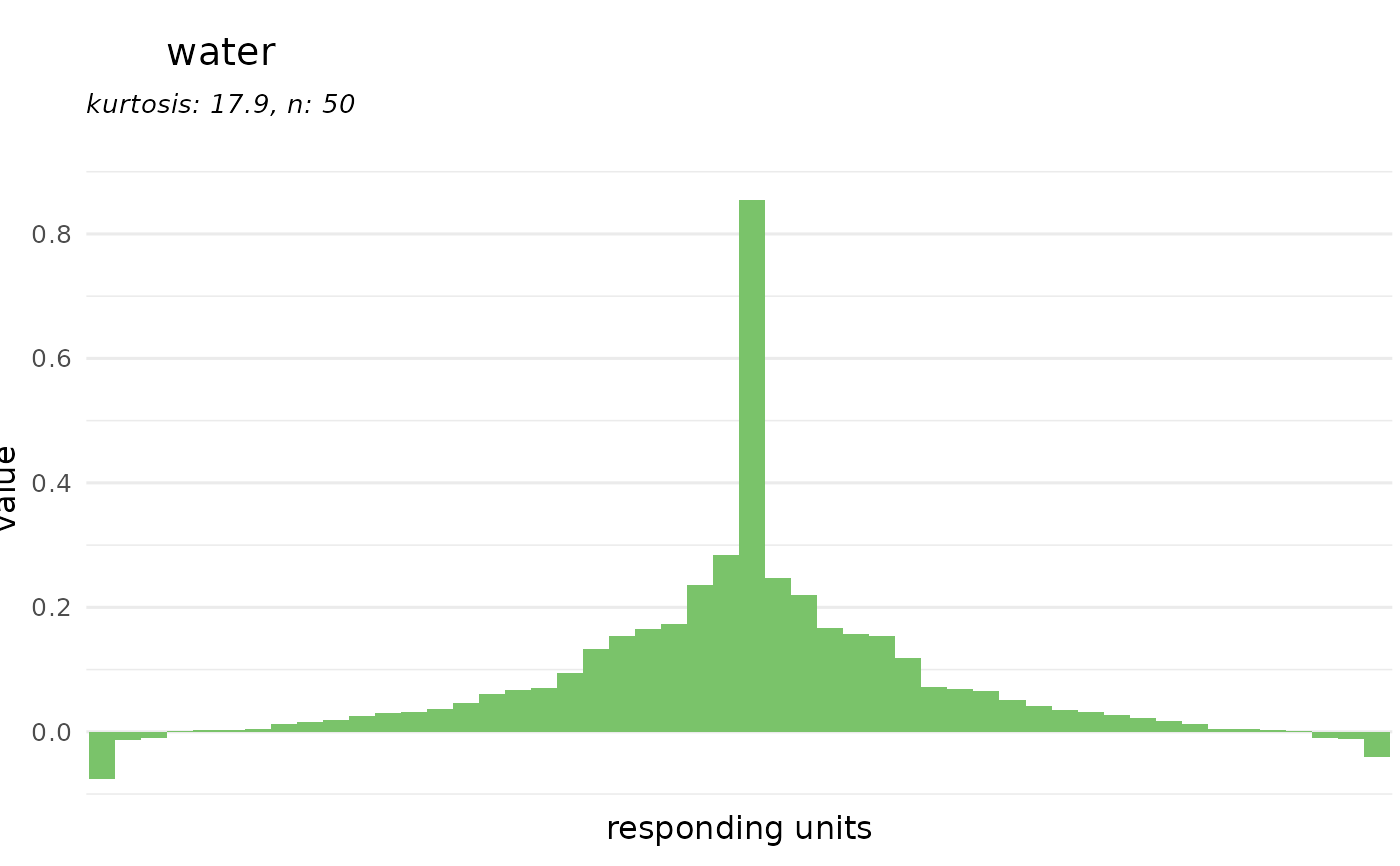# or for a receptor
dplot_tuningCurve(receptor = "Or22a")